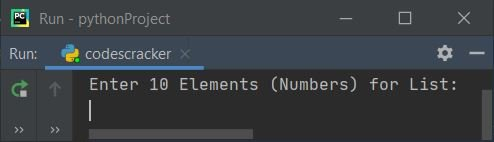# Python Program to Find the Smallest Number in a List

This article was created to cover some programs in Python that find and print the smallest number (element) in a list entered by the user. Here is a list of programs covered in this article:

• Find the smallest number in a list of 10 numbers.
• Find the smallest number in a list of n numbers.
• Find the smallest number in a list using the min() method.
• Find the smallest number in a list using the sort() method.

## Find the smallest number in a list of 10 numbers

The question is: write a Python program to find the smallest number in a list without using any predefined method. Here is its answer:

```nums = []
print("Enter 10 Elements (Numbers) for List: ")
for i in range(10):
nums.append(int(input()))
small = nums
for i in range(10):
if small>nums[i]:
small = nums[i]
print("\nSmallest Number is: ")
print(small)```

Here is the initial output of this program's sample run:Now supply the input, say 1, 2, 3, 4, 5, 6, 7, 8, 9, and 0 as ten numbers to find and print the smallest from the given list of numbers, as shown in the snapshot of the sample's run given below:## Find the smallest number in a list of n numbers

This is the modified version of the previous program. This program allows the user to define the size of the list before entering elements for it. The end is used in this program to skip the insertion of an automatic newline. str() converts any type of value to a string.

```nums = []
print(end="Enter the Size: ")
numsSize = int(input())
print(end="Enter " +str(numsSize)+ " Numbers: ")
for i in range(numsSize):
nums.append(int(input()))
small = nums
for i in range(numsSize):
if small>nums[i]:
small = nums[i]
print("\nSmallest Number = " + str(small))```

Here is its sample run with user input, with 5 as size and 5, 4, 3, 1, and 2 as five elements:## Find the smallest number in a list using min()

The question is: write a Python program to find the smallest number in a list using min(). Here is its answer:

```nums = list()
print(end="Enter the Size: ")
numsSize = int(input())
print(end="Enter " +str(numsSize)+ " Numbers: ")
for i in range(numsSize):
nums.append(int(input()))

print("\nSmallest Number = " + str(min(nums)))```

## Find the smallest number in a list using sort()

This program uses the sort() method to sort the list in ascending order (by default), and then, using list indexing, I've printed the smallest number in the given list, as shown in the program given below:

```nums = list()
print(end="Enter the Size: ")
numsSize = int(input())
print(end="Enter " +str(numsSize)+ " Numbers: ")
for i in range(numsSize):
nums.append(int(input()))
nums.sort()
print("\nSmallest Number = " + str(nums))```

Python Online Test

« Previous Program Next Program »